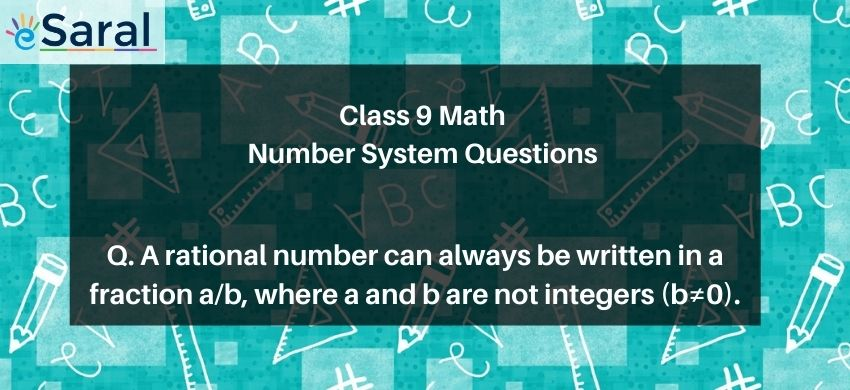Most Affordable JEE | NEET | 8,9,10 Preparation by Kota's Top IITian Doctor Faculties

# A rational number can always be written in a fraction $\frac{a}{b}$, where a and $b$ are not integers $(b \neq 0)$# Question : State True or False. A rational number can always be written in a fraction $\frac{\mathrm{a}}{\mathrm{b}}$, where a and $\mathrm{b}$ are not integers $(b \neq 0)$.

1. True
2. False
Solution :
The correct option is 2. False
A number that can always be written in the form of $\mathrm{p} / \mathrm{q}$, where $\mathrm{p}$ is any integer and $\mathrm{q}$ is a non-zero integer, is a rational number.
The given statement is false.